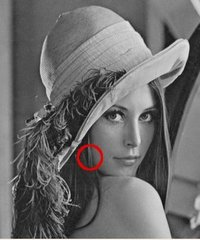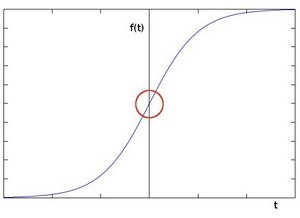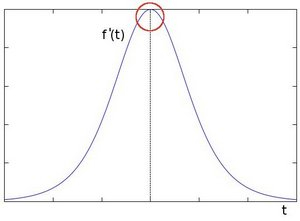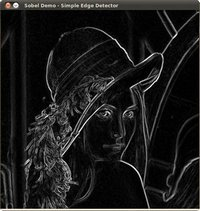## 理论#

1. 在之前的两个教程中，我们已经看到了卷积的应用实例。最重要的卷积之一是计算图像中的导数（或对它们的近似值）

2. 为什么图像中的导数计算可能是重要的？让我们设想一下，我们要检测图像中存在的边缘。比如说：你可以很容易地注意到，在一个边缘，像素强度的变化是很明显的。一个表达变化的好方法是使用导数。梯度的高变化表示图像中的一个重大变化。

3. 为了更加形象，让我们假设我们有一个一维图像。在下面的图中，一个边缘由强度的 “跳跃 “来表示：4. 如果我们取第一个导数，可以更容易地看到边缘的 “跳跃”（实际上，这里出现的是一个最大值）：5. 因此，从上面的解释中，我们可以推断出，检测图像中的边缘的方法可以通过定位梯度高于其邻居的像素位置（或者概括地说，高于一个阈值）来进行。

### Sobel 算子#

1. Sobel算子是一个离散的微分算子，它计算图像强度函数的梯度的近似值。
2. Sobel算子结合了Gaussian smoothing和微分。

### Formulation#

1. 我们计算两个导数：
1. 水平变化：这是通过用奇数大小的内核 $G_x$ 对 $I$ 进行卷积计算的。例如，对于内核大小为 $3$ ， $G_x$ 将被计算为： $$G_x=\begin{bmatrix} -1 & 0 & +1\\ -2 & 0 & +2\\ -1 & 0 & +1 \end{bmatrix} * I$$
2. 垂直变化：这是通过用奇数大小的内核 $G_y$ 对 $I$ 进行卷积计算的。例如，对于内核大小为 $3$ ， $G_y$ 将被计算为： $$G_y = \begin{bmatrix} -1 & -2 & -1\\ 0 & 0 & 0\\ +1 & +2 & + 1 \end{bmatrix} * I$$
2. 在图像的每一点上，我们通过结合上述两个结果计算出该点的梯度近似值： $$G = \sqrt{G_{x}^{2}+G_{y}^{2}}$$ 有时会使用以下更简单的方程式： $$G = |G_x|+|G_y|$$

## Code#

### Explanation#

#### 变量声明#

// First we declare the variables we are going to use
Mat image,src, src_gray;
const String window_name = "Sobel Demo - Simple Edge Detector";
int ksize = parser.get<int>("ksize");
int scale = parser.get<int>("scale");
int delta = parser.get<int>("delta");
int ddepth = CV_16S;


#### 加载图像#

String imageName = parser.get<String>("@input");
// As usual we load our source image (src)
// Check if image is loaded fine
if( image.empty() )
{
printf("Error opening image: %s\n", imageName.c_str());
return EXIT_FAILURE;
}


#### 消除噪声#

// Remove noise by blurring with a Gaussian filter ( kernel size = 3 )
GaussianBlur(image, src, Size(3, 3), 0, 0, BORDER_DEFAULT);


#### 灰度化#

// Convert the image to grayscale
cvtColor(src, src_gray, COLOR_BGR2GRAY);


#### Sobel 算子#

Mat grad_x, grad_y;
Sobel(src_gray, grad_x, ddepth, 1, 0, ksize, scale, delta, BORDER_DEFAULT);
Sobel(src_gray, grad_y, ddepth, 0, 1, ksize, scale, delta, BORDER_DEFAULT);

• 我们计算 $x$ 和 $y$ 方向的 “导数”。为此，我们使用函数Sobel()，如下所示。该函数需要以下参数:
• src_gray：在我们的例子中，输入的图像。这里是CV_8U
• grad_x / grad_y：输出图像。
• ddepth：输出图像的深度。我们将其设置为CV_16S以避免溢出。
• x_order： $X$ 方向上的导数顺序。
• y_order: $Y$ 方向的导数顺序。
• scaledeltaBORDER_DEFAULT：我们使用默认值。

#### 转换为CV_8U图像#

// converting back to CV_8U


#### 梯度#

addWeighted(abs_grad_x, 0.5, abs_grad_y, 0.5, 0, grad);


## 结果#https://docs.opencv.org/4.5.5/d2/d2c/tutorial_sobel_derivatives.html